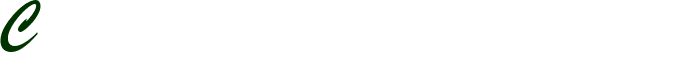# 456

456

Description  Details

Discipline Statistics

Assignment type : Other types

Description

IHP 525 Module Three Problem Set

1. What is the probability of being born on:
2. a) February 28?
3. b) February 29?
4. c) February 28 or February 29?
5. A patient newly diagnosed with a serious ailment is told he has a 60% probability of surviving 5 or more years. Let us assume this statement is accurate. Explain the meaning of this statement to someone with no statistical background in terms he or she will understand.
6. A lottery offers a grand prize of \$10 million. The probability of winning this grand prize is 1 in 55 million (about 1.8×10-8). There are no other prizes, so the probability of winning nothing = 1 – (1.8×10-8) = 0.999999982. The probability model is:

Winnings (X) 0 \$10 x 106

P(X = xi) 0.999999982 1.8 x 10-8

1. a) What is the expected value of a lottery ticket?
2. b) Fifty-five million lottery tickets will be sold. How much does the proprietor of the lottery need to charge per ticket to make a profit?
3. Suppose a population has 26 members identified with the letters A through Z.
4. a) You select one individual at random from this population. What is the probability of selecting individual A?
5. b) Assume person A gets selected on an initial draw, you replace person A into the sampling frame, and then take a second random draw. What is the probability of drawing person A on the second draw?
6. c) Assume person A gets selected on the initial draw and you sample again without replacement. What is the probability of drawing person A on the second draw?
7. Let A represent cat ownership and B represent dog ownership. Suppose 35% of households in a population own cats, 30% own dogs, and 15% own both a cat and a dog. Suppose you know that a household owns a cat. What is the probability that it also owns a dog?
8. What is the complement of an event?
9. Accidents occur along a 5-mile stretch of highway at a uniform rate. The following “curve” depicts the probability density function for accidents along this stretch:
10. a) What is the probability that an accident occurred in the first mile along this stretch of highway?
11. b) What is the probability that an accident did not occur in the first mile?
12. c) What is the probability that an accident occurred between miles 2.5 and 4?
13. Suppose there were 4,065,014 births in a given year. Of those births, 2,081,287 were boys and 1,983,727 were girls.
14. a) If we randomly select two women from the population who then become pregnant, what is the probability both children will be boys?
15. b) If we randomly select two women from the population who then become pregnant, what is the probability that the first woman’s child will be a boy and the second woman’s child will be a boy?
16. c) If we randomly select two women from the population who then become pregnant, what is the probability that both children will be boys given that at least one child is a boy?
17. Explain the difference between mutually exclusive and independent events.
18. Suppose a screening test has a sensitivity of 0.80 and a false-positive rate of 0.02. The test is used on a population that has a disease prevalence of 0.007. Find the probability of having the disease given a positive test result.

Format MLA

Volume of 2 pages (550 words)

Type of service: Custom writing## You Need a Professional Writer To Work On Your Paper?## Do you have an Urgent Assignment?;
BestQualityExperts

Disclaimer: The services that we offer are meant to help the buyer by providing a guideline.
The product provided is intended to be used for research or study purposes only.